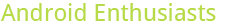## distribution of up and down votes

0

(click on this box to dismiss)Q&A about the site for enthusiasts and power users of the Android operating system

``````with p as (
select id
from PostsWithDeleted
where PostTypeId = 2
)   ,a as (
select p.id,
sum(case when v.votetypeid = 2 then 1 else 0 end) as upvotes,
sum(case when v.votetypeid = 3 then 1 else 0 end) as downvotes
on p.id = v.PostId
group by p.id
), m as (
count(id) as cnt,
case when upvotes = 0 then 'up = 0'
when upvotes = 1 then 'up = 1'
when upvotes <= 5 then '2 <= up <= 5'
when upvotes <= 10 then '6 <= up <= 10'
else '11 <= up'
end as part
from a
), sm as (
select part, sum(cnt) as vsum
from m
group by part
),  pm as (
m.part,
sum(cnt)  as  psum
from m
)Hold tight while we fetch your results